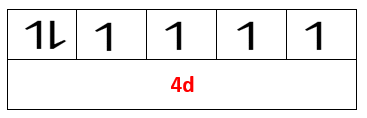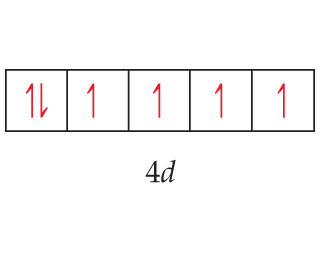# Problem: The orbital diagram that follows shows the valence electrons for a 2+ ion of an element. What is the element?

###### FREE Expert Solution

We are asked to find the identity of the +2 ion for the given valence electron configuration:Recall that every single element in the periodic table has a corresponding atomic number.

The atomic number of an element is always equal to the number of protons of that element. And when the element is neutral (charge = 0), the number of protons is equal to the number of electrons.

Neutral element:          Atomic number = # protons = # of electrons

79% (55 ratings)###### Problem Details

The orbital diagram that follows shows the valence electrons for a 2+ ion of an element.What is the element?